## Books : reviews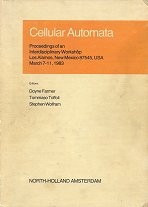### J. Doyne Farmer, Tommaso Toffoli, Stephen Wolfram, eds.Cellular Automata: Proceedings of an Interdisciplinary Workshop, Los Alamos March 7-11, 1983 (Physica D 10). North Holland. 1984

#### Contents

Norman H. Margolus. Physics-like models of computation. Physica 10D. 1984
Stephen Wolfram. Universality and complexity in Cellular Automata. 1984
Douglas A. Lind. Applications of ergodic theory and sofic systems to Cellular Automata. 1984
Michael S. Waterman. Some applications of information theory to Cellular Automata. 1984
Peter Grassberger. Chaos and diffusion in deterministic Cellular Automata. 1984
Encode the 1D CA state …s-2s-1s0s1s2… as the 2D point (0.s0s1s2…, 0.s-1s-2…) • "similar" states are "close" in space (with a bias to similarity near cell c0, consistent with the Cantor set topology explained in [Toffoli 1984a] below) •  time evolution is a trajectory in this space • some CA rules have dynamics that exhibit an "attractor"-like structure (although not completely identical) in this space
T. E. Ingerson, R. L. Buvel. Structure in asynchronous Cellular Automata. 1984
asynchronous 1D CA investigations • random, and own clock • these models are more "natural" •  some self-organisin behaviour of synchronous 1D CAs comes from the synchronisation • some further interesting behaviour appears with asynchronous models
Stephen J. Willson. Growth rates and fractional dimensions in Cellular Automata. 1984
R. Wm. Gosper. Exploiting regularities in large cellular spaces. 1984
Optimisation algorithm for 2D CAs, using quadrant decomposition of cellular space, cacheing of results, and hashing to find previously used quadrants
Gerard Y. Vichniac. Simulating physics with Cellular Automata. 1984
CAs exactly computable models, and non-numerical simulations • a naive CA implementation of a spin glass gives poor results
Tommaso Toffoli. Cellular Automata as an alternative to (rather than an approximation of) differential equations in modeling physics. 1984
why CAs are appropraite for direct modelling of physical systems • Cantor set topology of infinite CAs
Stephen M. Omohundro. Modelling Cellular Automata with partial differential equations. 1984
Christopher G. Langton. Self-reproduction in Cellular Automata. 1984
requirement that self-replicator be a universal constructor is too strong: natural self-replicators (organisms!) aren't universal constructors • relax therequirement simply to: the "self-replicating" configuration must treat its stored information both as interpreted instructions and uninterpreted data • adaptation of Codd's 1968 universal constructor, with a different transition rule • states comprise instructions for constructing a new loop • instructions travel around the loop, memory - uninterpreted • instructions construct new loop - interpreted
Stuart A. Kauffman. Emergent properties in random complex automata. 1984
N cells with boolean state, each getting input from K other randomly chosen cells, combined by a randomly chosen boolean function •  K = 2 dynamics properties : number of states = 2N, yet cycle length, number of distinct cycles (basins of attraction) ~ N1/2; cycles relatively stable to small perturbations •  canalising rules, and forcing structures - subgraphs that "crystallise" at their canalised values - and so partition the remaining graph into isolated subclusters •  as simple models of geneetic regulatory networks
Christian Burks, J. Doyne Farmer. Towards modeling DNA sequences as automata. 1984
Steven A. Smith, Richard C. Watt, Stuart R. Hameroff. Cellular Automata in cytoskeletal lattices. 1984
Forrest L. Carter. The molecular device computer: point of departure for large scale Cellular Automata. 1984
Tommaso Toffoli. CAM: a high-performance Cellular-Automaton Machine. 1984
support for "watching 2D CA evolution •  sequential processing, special-purpose hardware •  256x256 array of cells, periodic (toroidal) boundary conditions •  each cell with up to 256 states •  60 timesteps per second display
Kendall Preston Jr. Four-dimensional logical transforms: data processing by Cellular Automata. 1984
W. Daniel Hillis. The Connection Machine: a computer architecture based on Cellular Automata. 1984
James P. Crutchfield. Space-time dynamics in video feedback. 1984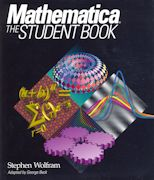### Stephen Wolfram, George Beck.Mathematica: the Student Book. Addison-Wesley. 1990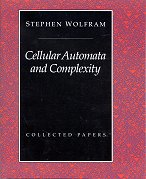### Stephen Wolfram, ed.Cellular Automata and Complexity: collected papers. Addison-Wesley. 1994

#### Contents

Universality and complexity in Cellular Automata. 1984
Statistical mechanics of Cellular Automata. Rev.Mod.Phys 55:601-644. 1983
Stephen Wolfram, Olivier Martin, Andrew M. Odlyzko. Algebraic properties of Cellular Automata. Comm.Math.Phys 93:219-258. 1984
Computation theory of Cellular Automata. Comm.Math.Phys 96:15-57. 1984
Undecidability and intractability in theoretical physics. Phys.Rev.Lett 54:735-738. 1985
Norman H. Packard, Stephen Wolfram. Two dimensional Cellular Automata. J.Stat.Phys 38:901-946. 1985
Origins of randomness in physical systems. Phys.Rev.Lett 55:449-452. 1985
Stephen Wolfram, James B. Salem. Thermodynamics and hydrodynamics of Cellular Automata. 1985
Random sequence generation by Cellular Automata. Adv.Appl.Math 7:123-169. 1986
Approaches to complexity engineering. Physica D 22:385-399. 1986
Minimal Cellular Automaton approximations to continuum systems. 1986
Cellular Automaton fluids: basic theory. J.Stat.Phys 45:471-526. 1986
Cellular Automata. Los Alamos Science 9:2-21. 1983
Computers in science and mathematics. Scientific American 251:188-203. 1984
Geometry of binomial coefficients. American Mathematical Monthly 91:566-571. 1984
Twenty problems in the theory of Cellular Automata. Physica Scripta T9:170-183. 1985
Cryptography with Cellular Automata. Crypto'85, LNCS 218:429-432. Springer. 1986
Complex systems theory. 1988
Cellular Automaton supercomputing. 1988
Tables of Cellular Automaton properties. 1986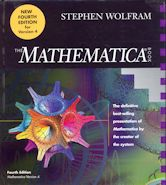### Stephen Wolfram.The Mathematica Book: 4th edn. CUP. 1999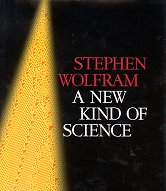Other reviews: